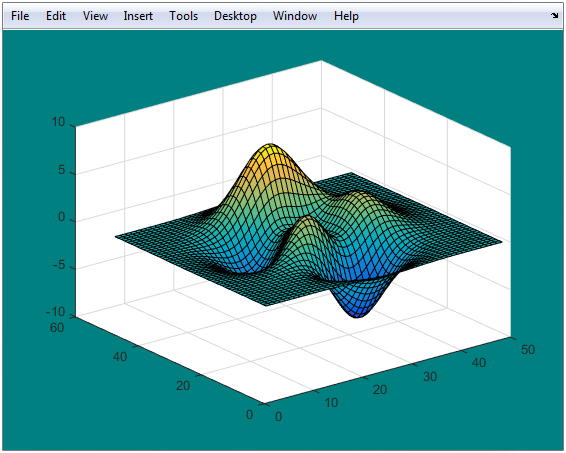Main Content

# gcf

Current figure handle

## Syntax

``fig = gcf``

## Description

example

````fig = gcf` returns the current figure handle. If a figure does not exist, then `gcf` creates a figure and returns its handle. You can use the figure handle to query and modify figure properties. For more information, see Figure Properties.```

## Examples

collapse all

Set the background color and remove the toolbar for the current figure. Use the `gcf` command to get the current figure handle.

```surf(peaks) fig = gcf; % current figure handle fig.Color = [0 0.5 0.5]; fig.ToolBar = 'none';```## More About

collapse all

### Current Figure

The current figure is the target for graphics output. It is the figure window in which graphics commands such as `plot`, `title`, and `surf` draw their results. It is typically the last figure created or the last figure clicked with the mouse.

User interaction can change the current figure. If you need to access a specific figure, store the figure handle in your program code when you create the figure and use this handle instead of `gcf`.

## Tips

• To get the handle of the current figure without forcing the creation of a figure if one does not exist, query the `CurrentFigure` property on the root object.

```fig = get(groot,'CurrentFigure'); ```
MATLAB® returns `fig` as an empty array if there is no current figure.

## See Also

### Properties

Introduced before R2006a

Download ebook